Register for Maths, Science, English, Reasoning Olympiad Exams Register here | Book Free Demo for Live Olympiad Classes here | Check Olympiad Exam Dates here | Buy Practice Papers for IMO, IOM, HEO, IOEL etc here | Login here to participate in all India free mock test on every Saturday

# Arithmetic Progression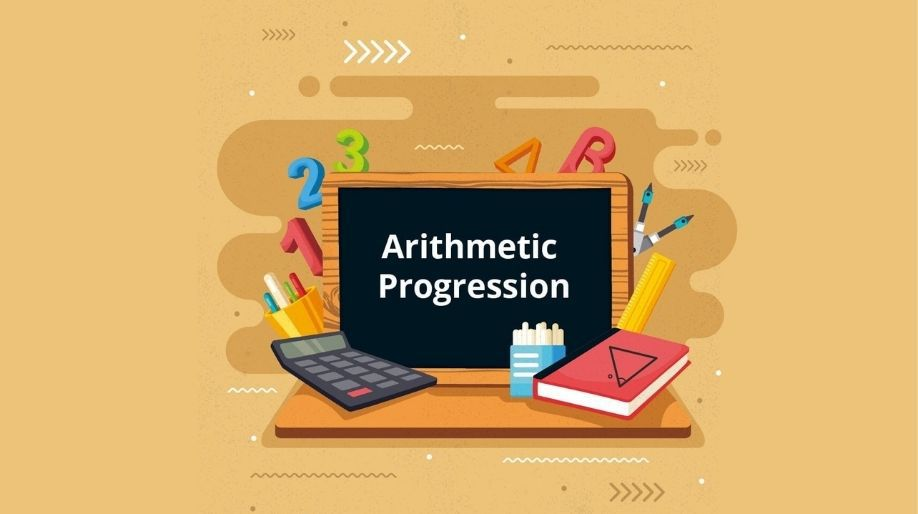Arithmetic Progression

If we observe then we may notice that there is some pattern in some things like 2,4,6,8 are all even numbers and the difference is same that is two. It means on adding 2 in each even number we get a new even number. Same things happen with odd numbers also. Like this there a lot of patterns. So, let’s learn more about the topic and patterns.

As the name suggest Arithmetic means adding or subtracting and progression means series.

A sequence of numbers such that the difference between the consecutive term is constant then the sequence is said to be in Arithmetic Progression.

For example 3,7,11,15,19,23. Here each term differs the previous term by 4 and since the difference is constant therefore it is a arithmetic progression.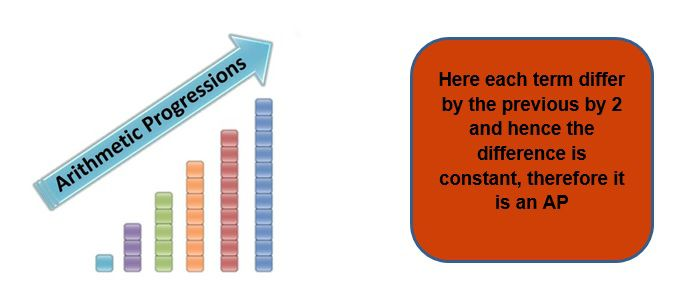• The constant difference is actually known as common difference and is denoted by d.
• The first term of an AP is denoted by a.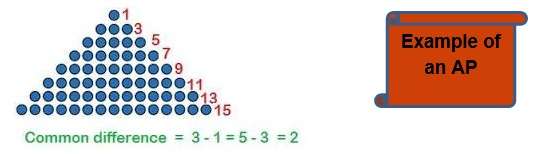Therefore the general form of an Arithmetic Progression is a, a+d, a+2d, a+3d, a+4d………….n times.

• Here we can notice that the common difference between each term is d.

Common Difference:

The common difference can be positive, negative or even zero.

• If the difference is positive then the AP is called increasing AP. For example 2, 12, 22, 32, 42, 52.
• If the difference is negative then the AP is called decreasing AP. For example 12, 10, 8, 6, 4.
• If the difference is zero then it is called as constant AP. For example 5, 5, 5, 5, 5, 5, 5, 5.

If we denote the first term by a1, second term by aand so on therefore the nth  term will be an. Then the AP hence formed will be a1, a2, a3, ……………..an.

So, a2-a1 = a3-a2 = a4-a3 ………..= an-an-1 = d.

Nth Term of an AP

It is not always possible to determine all the terms of an AP if it contains a lot of terms. For example if we have to determine the 1000th term of an AP of even nos then it is not that easy and also time taking.

Hence we generalize the result to find the nth term of an AP

Let a1, a2, a3, ……………..an, be an AP of n terms and here a1 is the first term and d is common difference.

a2 = a + d = a + (2-1)d

a3 = a+2d = a + (3-1)d

a4 = a+3d= a + (4-1)d

Similarly

General Term an =  a + (n-1)d

And the last term of AP l = a + (n-1)d if there are n term

Example1:- Find the 10th term of the AP 2,12, 22, 32….

Solution:- Here first term a = 2 and common difference d is

12-2 = 10 = d.

Using the formula and putting n=10 we get

a10 = 2+ (10-1)10 = 2 + 90 = 92.

Example 2:- Which term of the AP 15,10, 5, 0 … will be -40?

Solution:- Here a =15 and d = -5.

And here as we know that an = -40.

On putting the values in the formula we get

-40 = 15 + (n-1)(-5)

-55 = (n-1)(-5)

Therefore (n-1) = 11 and hence n = 12.

So the 12th term will be -40.

Sum of N terms of an AP

Let us consider an AP with first term a and common difference d

a, a+d, a+2d, a+3d, a+4d………………………a+(n-1)d.

Let S denotes the sum of the first n terms and therefore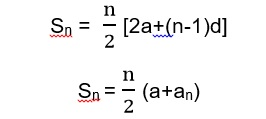• Here an important point is to remembered that an = Sn-Sn-1.

It means that the nth term of an AP is equal to the difference of its sum of nth and sum of (n-1)th term.

Example 3:- Find the sum of 10 terms of the AP -3, 1, 5, 9,13..?

Solution:-  Here a = -3 and d = 4

Therefore on putting the values in the formula we get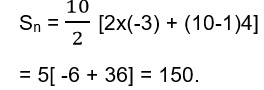Hence the sum of first 10 terms is 150.

Word Problems

Example 4:- Raman started working at a company at an annual salary of Rs 5000 per year and he received an annual increment of Rs 200 per year. After how many years his salary will be Rs 8000?

Solution:- Here the sequence will be 5000, 5200, 5400, 5800, 6000……….8000

Here the first term a =5000 and d=200, an = 8000

On using the formula and putting the values in it, we get

8000 = 5000 +(n-1)200

3000 = (n-1)200 and hence (n-1) = 15

Therefore n= 16 which means after 16 years Raman’s annual salary will be Rs 8000 per year.

Example 5:- A contract company has to pay penalty if the works get delayed. Rs 100 for first day, Rs 150 for second, Rs 200 for third and so on. Find out how much money the contractor has to pay if the work gets delayed by 30 days.

Solution:- The sequence formed will be 100 , 150, 200, 250 …

Here a=100 and d=50 and n=30

Therefore on putting the values in the formula we get,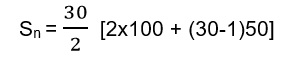Sn = 15[200+1450]

Sn = 15 x 1650 = 24750.

Hence the contractor has to pay Rs 24,750 as penalty for the delay of work for 30 days.

Practice Questions

Q1) Write the first four terms of the AP if

1. a= 3 and d=5 ii) a=12 and d=-2
• a=4 and d=-2 iv) a=10 and d= 10

Q2) Find a1, a2, a4  and d of the following AP

1. 10, 5, 0, -5,-10 ….. b) -20,-12,-4, 4…..

Q3) Find the nth term of the following AP

1. 5, 11, 17, 23, 29…….
2. 10, 15 ,20, 25 ……….

Q4) Determine the AP whose first term is 8 and 3rd term is 24.

Q5) How many two digit numbers are divisible by 5?

Q6) Find the sum first 200 even integers.

Recap

• The sequence having a common difference is known as AP.

• The general term is an = a+(n-1)d

• The common difference d can be positive or negative or zero also.
• The addition, subtraction, multiplication or division of two AP’s also results in an AP.

## Quiz for Arithmetic Progression

 Q.1 In the AP 4,7,10, the term 100 occurs at what position? a) 33 b) 40 c) 45 d) 32
 Q.2 If a, 2a+1, 5a are in AP, what is the common difference? a) 3 b) -1 c) 2 d) -2
 Q.3 In a bank loan scheme for Rs. 10000 to be paid over 5 years with an initial down payment Rs. 2000 and the interest at 5% P.A. What is the installment paid at last term? a) 3500 b) 2500 c) 4000 d) 2000
 Q.4 Sum of the series : 1+(1+1)+(1+1+1)+(1+1+1+1)… upto n-1 terms a) n(n+1)/2 b) n2/2 c) n(2n-1)/2 d) n(n-1)/2
 Q.5 Find the 11th term from the last term (towards the first term) of the AP : 11, 8, 5, . . ., – 61. a) -32 b) -31 c) -41 d) 0
 Q.6 Which of the following is a term of the list of numbers 7, 13, 19, 25, . . a) 308 b) 303 c) 305 d) 304
 Q.7 Find the max possible value of n:-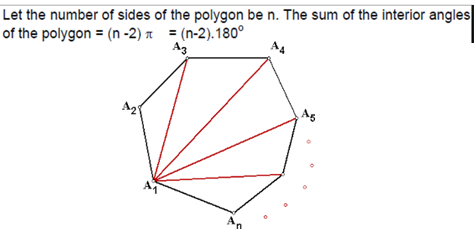a) 8 b) 9 c) 10 d) 16
 Q.8 Find the first term of an AP whose 8th and 12th terms are respectively 39 and 59. a) 4 b) 6 c) 8 d) 7
 Q.9 The common difference of the AP for which A18-A14=32 is _________. a) 8 b) -8 c) -4 d) 4
 Q.10 How many three digit numbers are divisible by 7? a) 128 b) 135 c) 130 d) 125